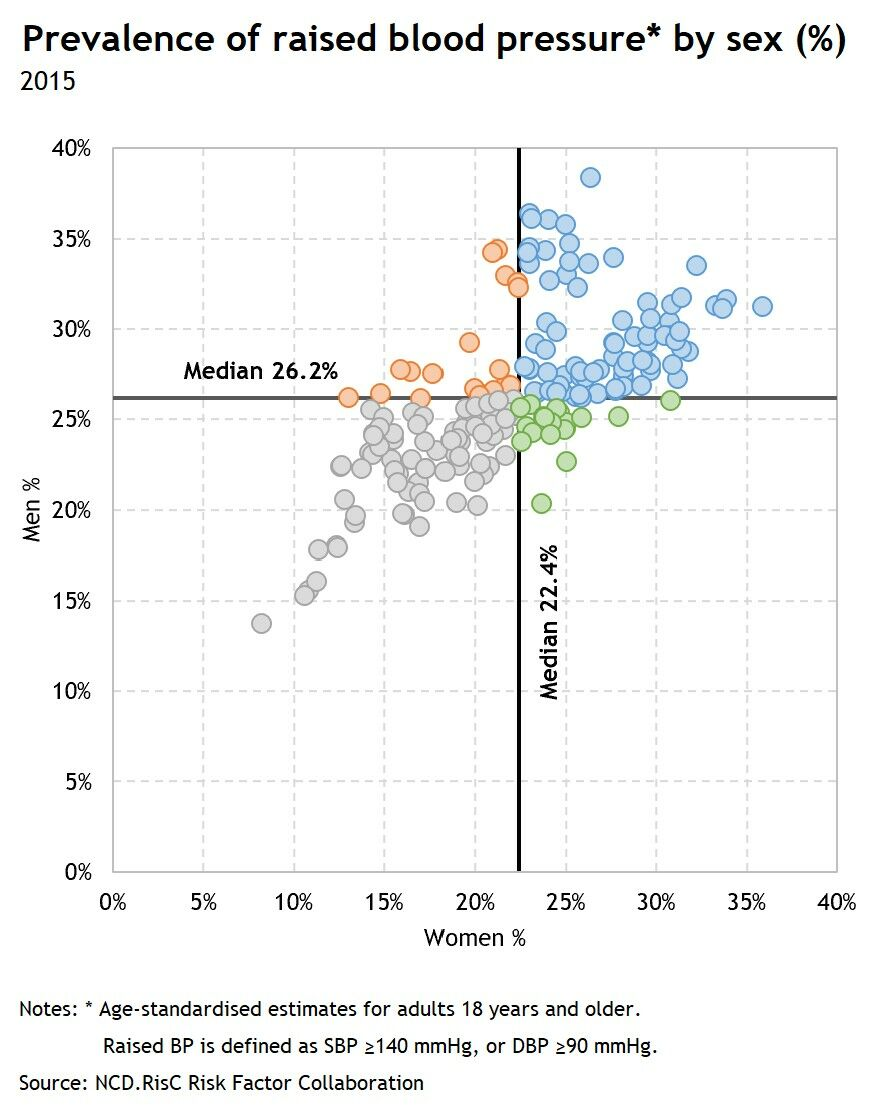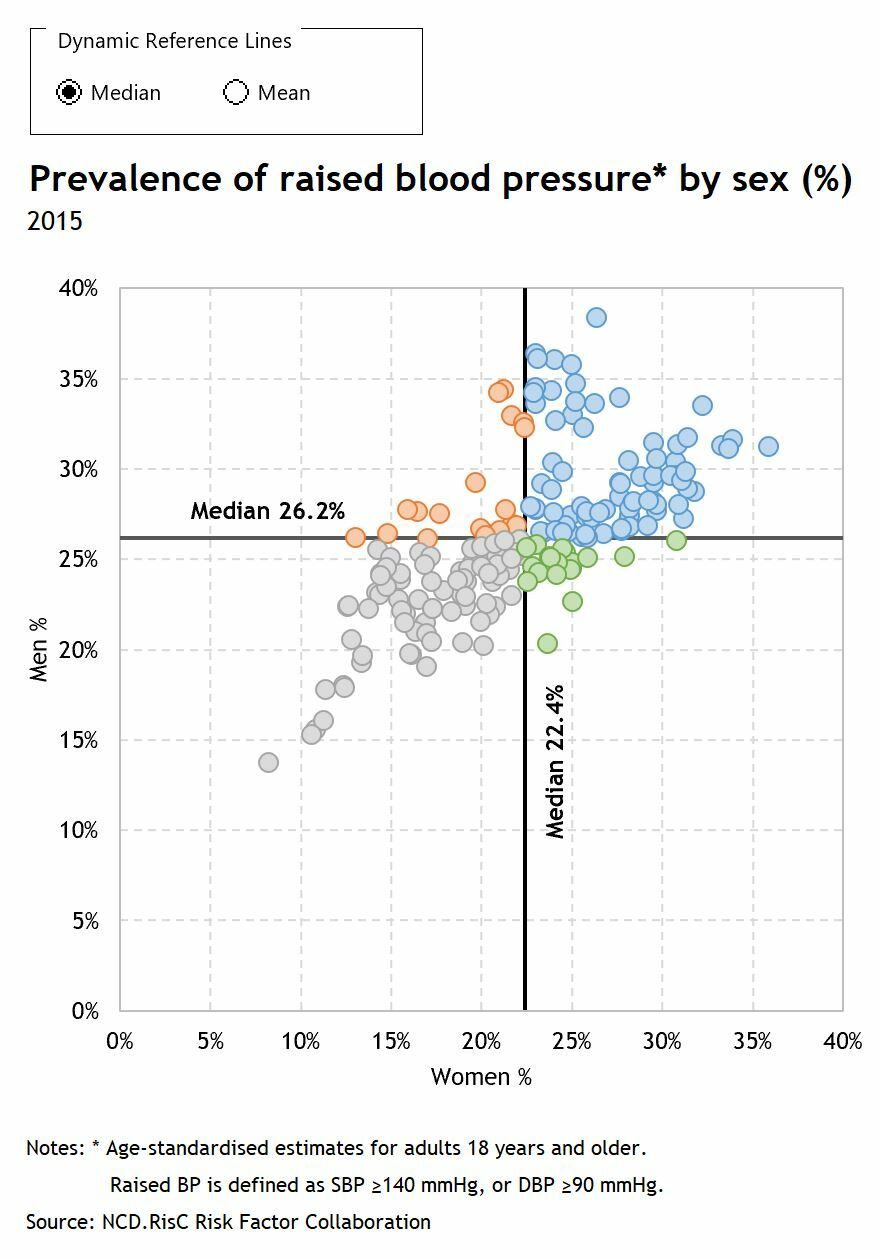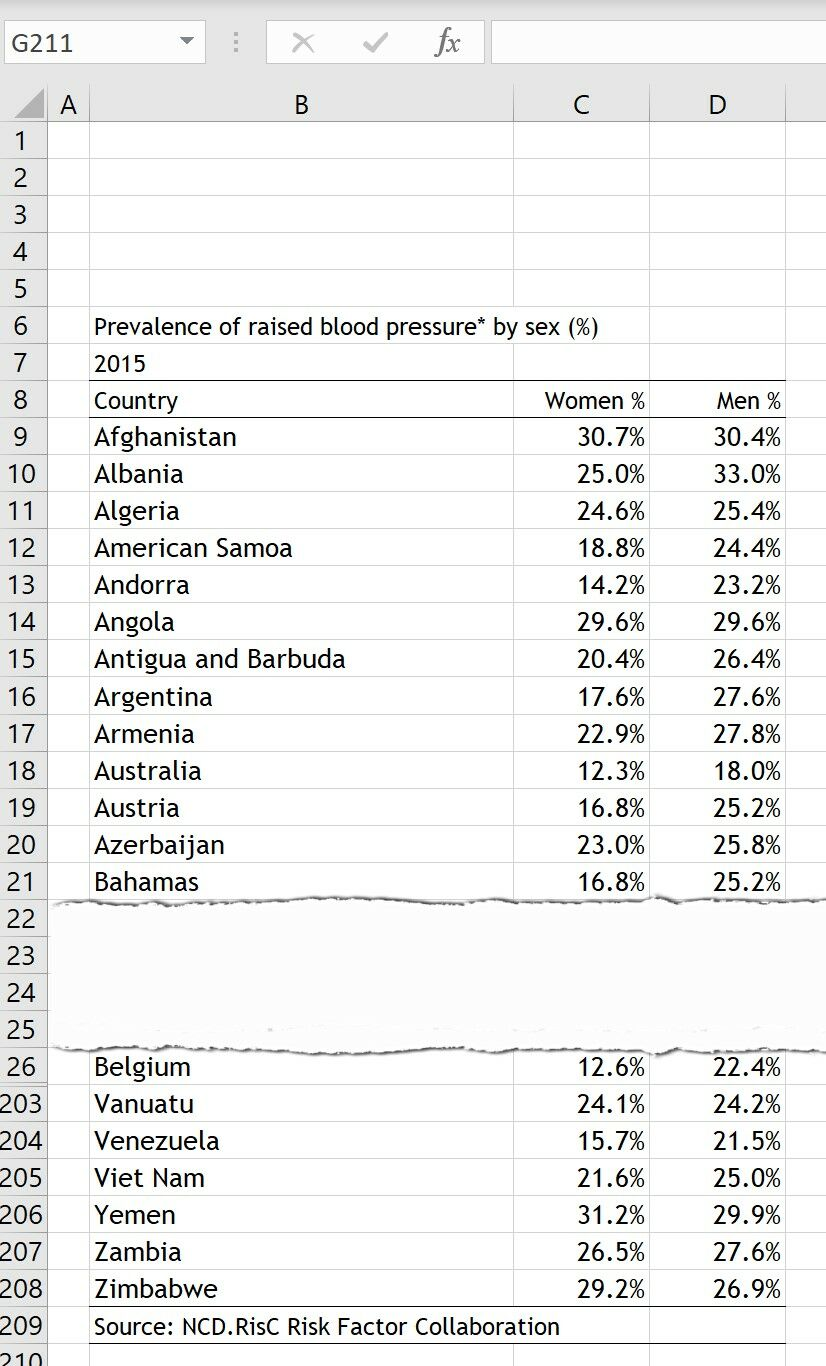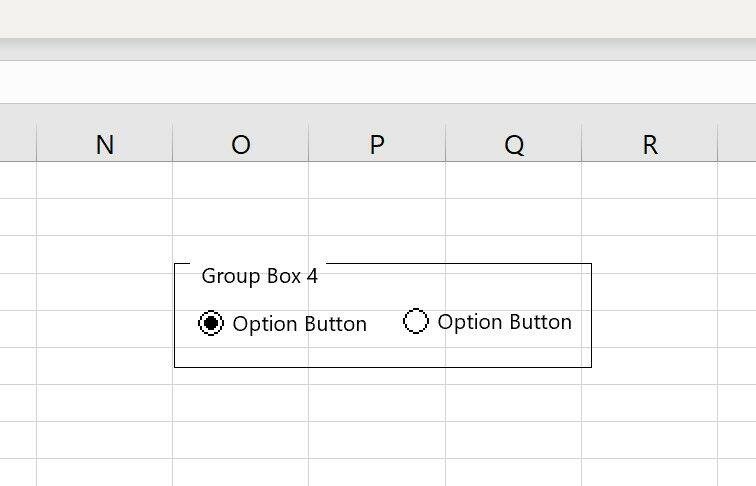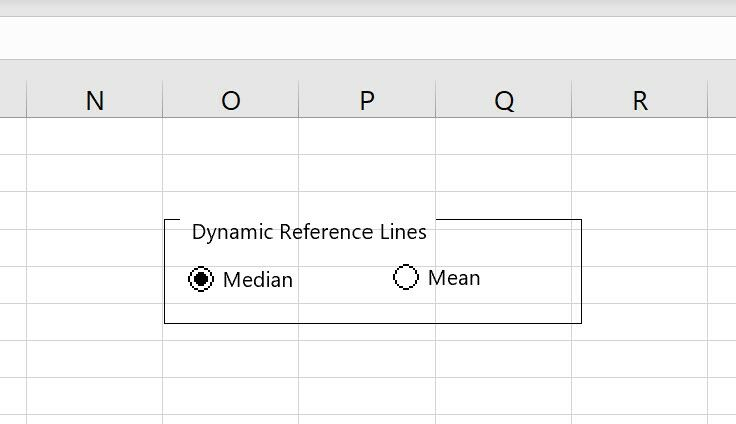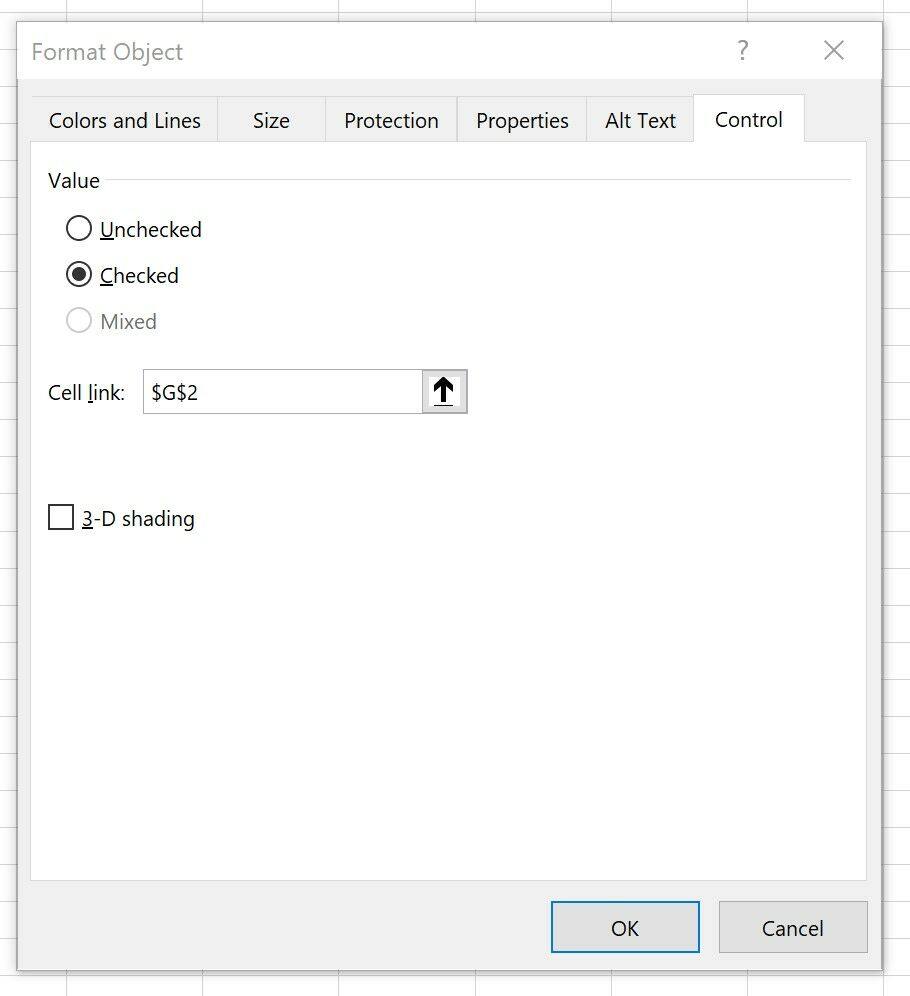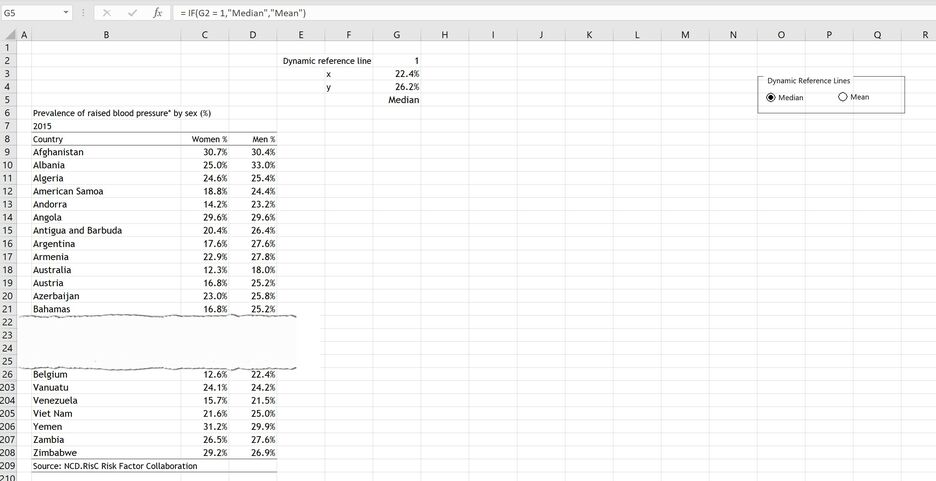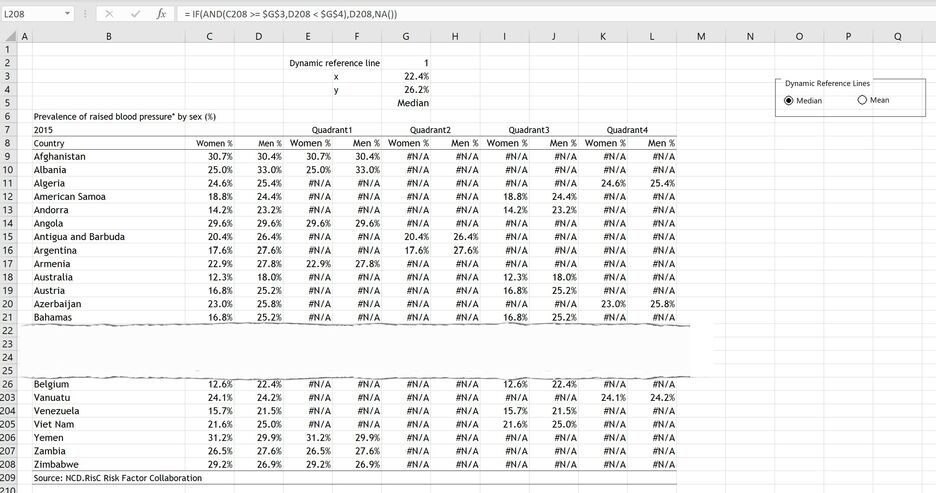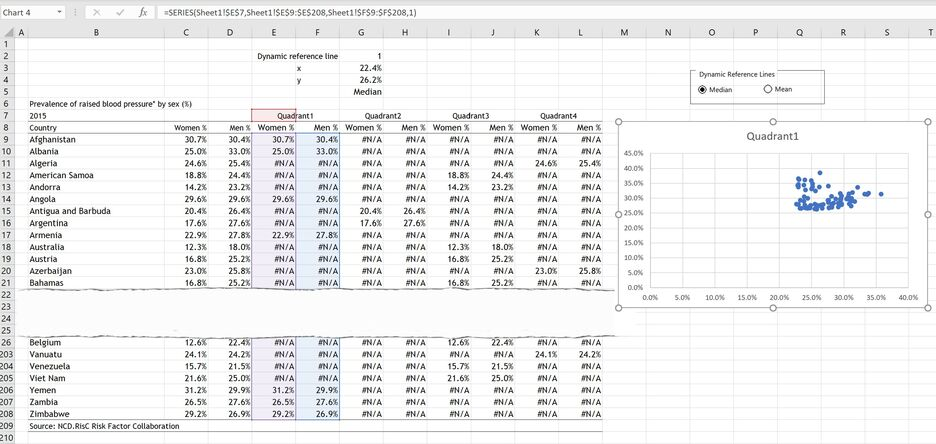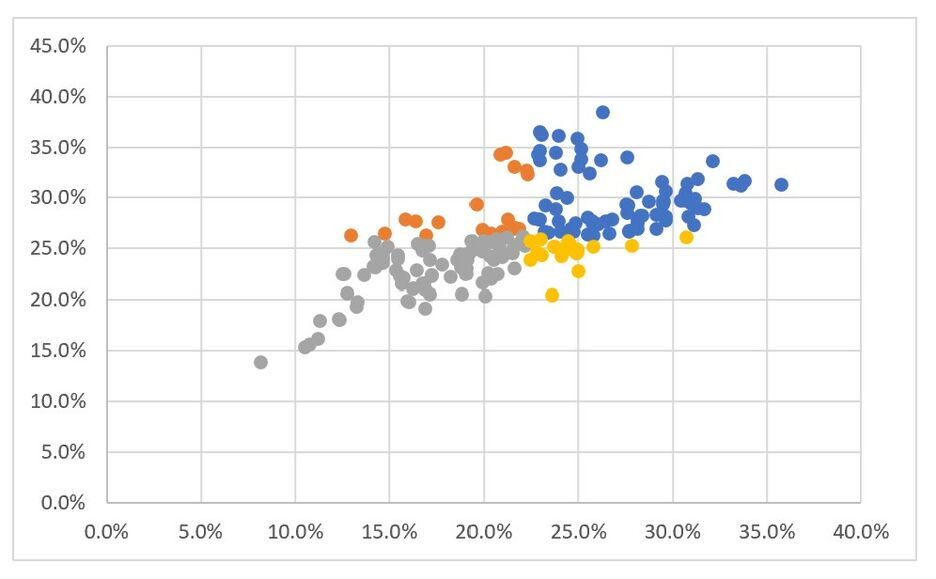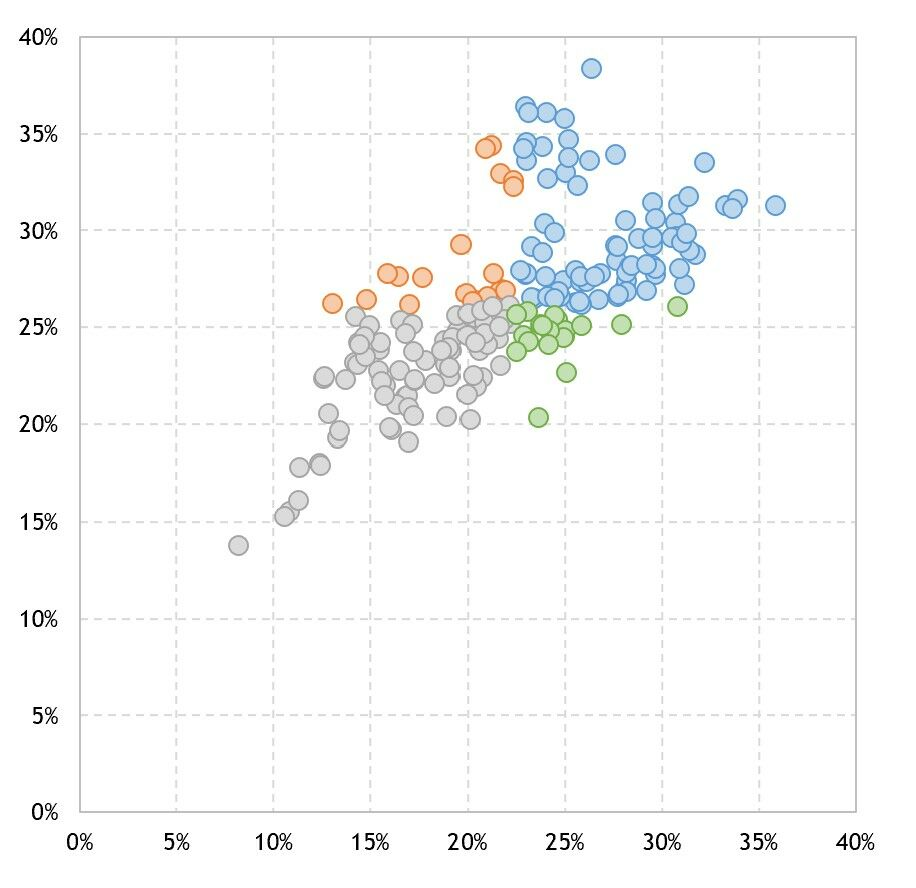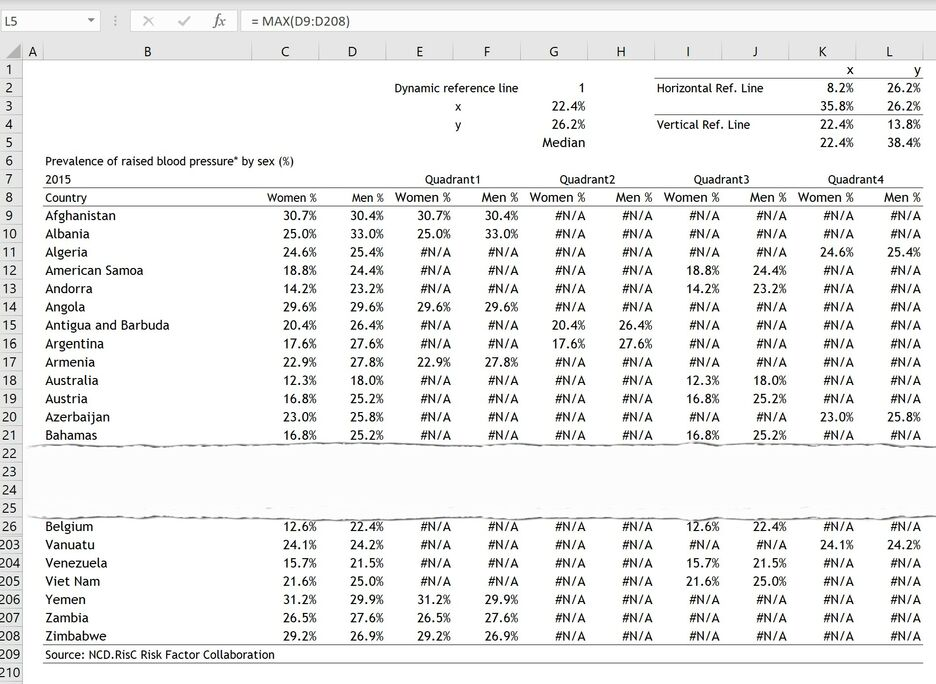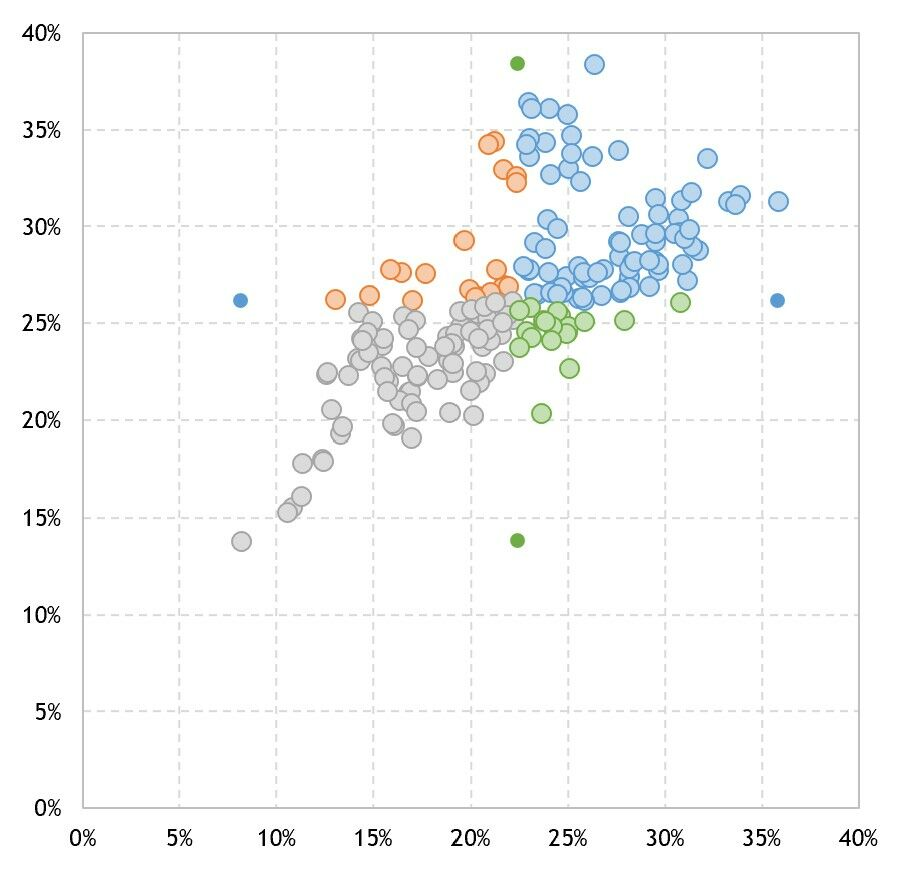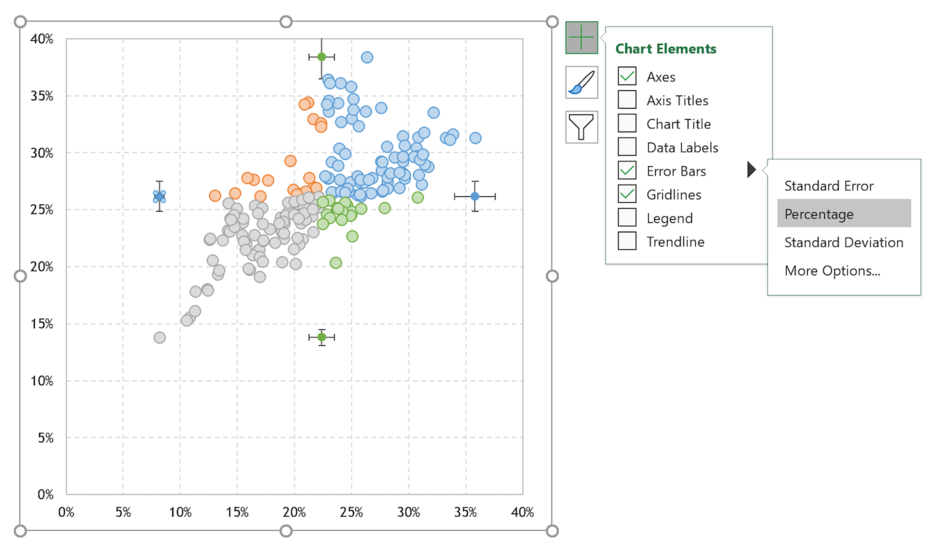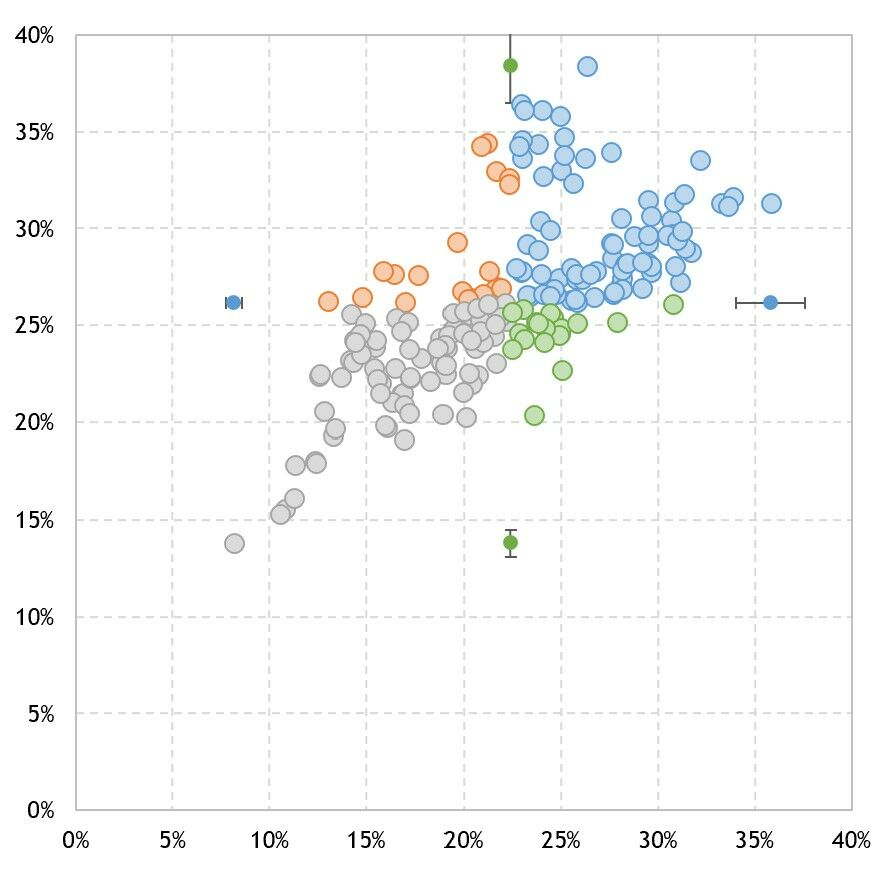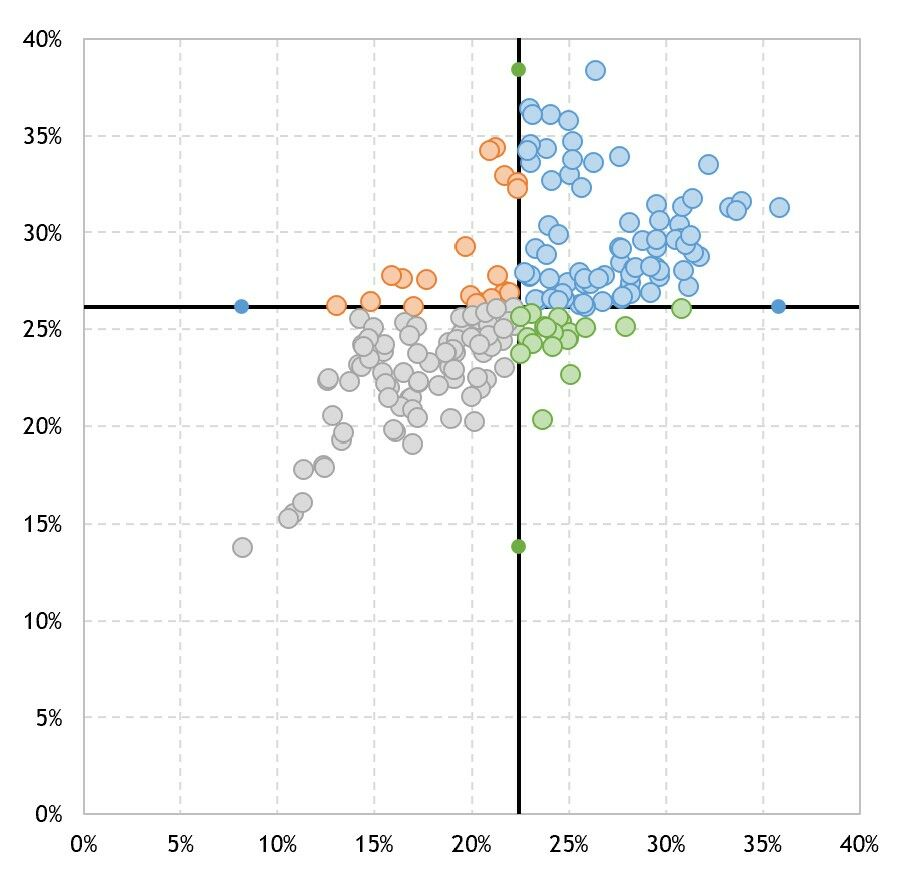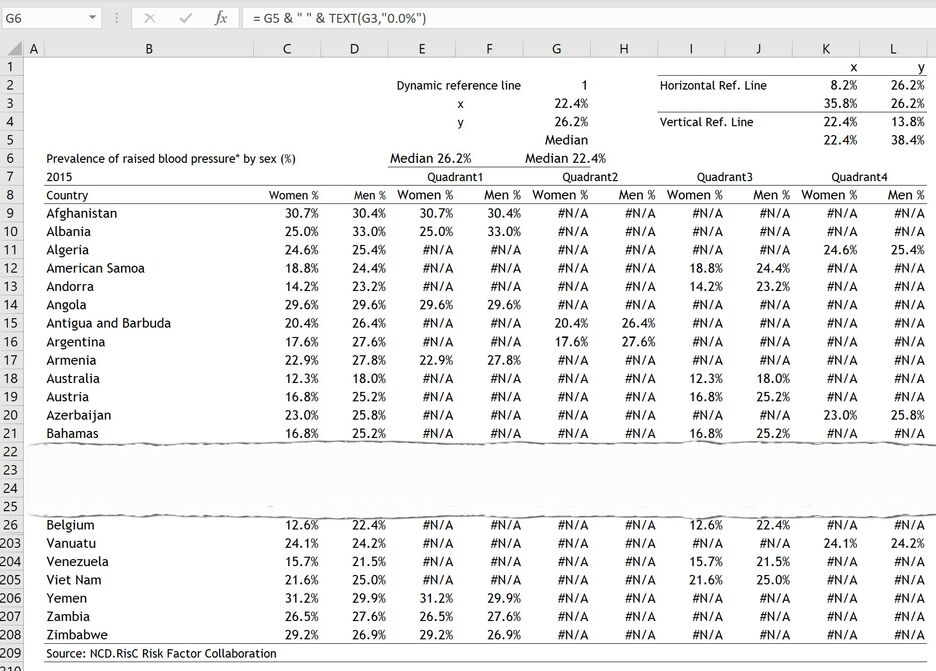• WORK
• TOOLS
• TRAINING
• VIEWS
• CONTACT

Views > How to create a scatterplot with dynamic reference lines in excel

Sometimes it is useful to add reference lines to charts to make comparisons easier. For example, the scatterplot below is divided into four meaningful quadrants by using the medians as reference lines. The chart provides the option to include reference lines for the mean and median. It also provides a mean to label reference lines to indicate what those lines represent. And Finally, it automatically splits the data into four data series—one for each quadrant—hence, allowing for different formatting options for each quadrant.

The data for the above chart is shown in the table below. It shows the prevalence of raised blood pressure among men and women in 200 countries and territories for the year 2015. The source of the data is from NCD.RisC.

1. On the Developer tab, in the Controls group, click on the Insert icon and then select the Group Box (Form Control) from the Form Controls dropdown menu.
2. Next, with the mouse, draw a rectangle on the worksheet to insert the Group Box.
3. Next, on the Developer tab, in the Controls group, click on the Insert icon and then select the Option Button (Form Control) from the Form Controls dropdown menu.
4. Next, with the mouse, draw a rectangle on the worksheet—inside the previously added Group Box—to insert the Option Button.
5. Repeat the above process and add second Option Button within the Group Box. The worksheet should look like this:

6. Edit the title of the Group Box to read “Dynamic Reference Lines” and the title of the first Option Button to read “Median” and that of the second Option Button to read “Mean”. The worksheet should look like this:

# HOW TO CREATE A SCATTERPLOT WITH DYNAMIC REFERENCE LINES IN EXCEL

HOW TO CREATE A SCATTERPLOT WITH DYNAMIC REFERENCE LINES IN EXCEL

7. Right-click the “Median” Option Button and then, on the shortcut menu, click Format Control.
8. In the Format Object dialog box let the Cell link refer to G2, as per the screen shot below:

9. Click the Ok button to close the Format Object dialog box. Like this I created a link between cell G2 and the two Option Buttons—where cell G2 has a value of 1 if Median is selected and a value of 2 if Mean is selected.
10. Next, lets calculate the thresholds for creating the 4 quadrants by introducing the following formulas in the respective cells:
G3 --> = IF(G2 = 1, MEDIAN(C9:C208),AVERAGE(C9:C208))
G4 --> = IF(G2 = 1, MEDIAN(D9:D208),AVERAGE(D9:D208))
G5 --> = IF(G2 = 1,"Median","Mean")
The worksheet should look like this:

11. Next, I need to create the setup to split the original data into four different series depending in which quadrant the data point falls. To do this add the following formulas in the respective cells:
E9 --> = IF(AND(C9 >= \$G\$3,D9 >= \$G\$4),C9,NA())
F9 --> = IF(AND(C9 >= \$G\$3,D9 >= \$G\$4),D9,NA())
G9 --> = IF(AND(C9 < \$G\$3,D9 >= \$G\$4),C9,NA())
H9 --> = IF(AND(C9 < \$G\$3,D9 >= \$G\$4),D9,NA())
I9 --> = IF(AND(C9 < \$G\$3,D9 < \$G\$4),C9,NA())
J9 --> = IF(AND(C9 < \$G\$3,D9 < \$G\$4),D9,NA())
K9 --> = IF(AND(C9 >= \$G\$3,D9 < \$G\$4),C9,NA())
L9 --> = IF(AND(C9 >= \$G\$3,D9 < \$G\$4),D9,NA())
12. Next, select the range E9:L9 then drag the handle to copy the formulas into cells E10:L208. Add column headers and the data source should look like this:

13. Highlight the range E9:F208 and then click Insert > Scatter > Scatter. The chart should look like this:

14. Right-click the chart and then, on the shortcut menu, click Select Data.
15. In the Select Data Source dialog box, select the Series1 data series and then click the Edit button under Legend Entries (Series).
16. In the Edit Series dialog box, edit the Series name to refer to cell E7. Click on the Ok button to close the Edit Series dialog box.
17. Next, In the Select Data Source dialog box, add a new data series by clicking the Add button under Legend Entries (Series).
18. In the Edit Series dialog box, refer to cell G7 in the Series name edit box. Next, edit the Series X values to include cells G9:G208 and the Series Y values to include cells H9:H208. Click on the Ok button to close the Edit Series dialog box.
19. Repeat the same process to add Quadrant 3 and Quadrant 4 data series to the chart. The chart should look like this:

20. Lets do some tidying up. Format the border of the Plot Area to Solid line with grey color.
21. Change the horizontal and vertical gridlines Dash type to Dash.
22. Set the chart font to Trebuchet MS, its color to black and font size to 9.
23. Turn off the border of the Chart Area by setting the Border to No line.
24. For each Quadrant data series, set the Marker size to 7, and change the Line and Fill color to something of your choice.
25. Set the Maximum Bound of the horizontal and vertical axis to 40% and change the Decimal places to 0.
26. Resize the chart so that its vertical and horizontal dimensions are equal. The chart should look like this:

27. Next, I need to define the vertical and horizontal reference lines. To do that introduce the below formulas in the respective cells:
K2 --> = MIN(C9:C208)
K3 --> = MAX(C9:C208)
K4 --> = G3
K5 --> = G3
L2 --> = G4
L3 --> = G4
L4 --> = MIN(D9:D208)

L5 --> = MAX(D9:D208)
The spreadsheet should look like this:

28. Right-click the chart and then, on the shortcut menu, click Select Data.
29. Next, in the Select Data Source dialog box, add a new data series by clicking the Add button under Legend Entries (Series).
30. In the Edit Series dialog box, refer to cell I2 in the Series name edit box. Next, edit the Series X values to include cells K2:K3 and the Series Y values to include cells L2:L3. Click on the Ok button to close the Edit Series dialog box.
31. Repeat the same process to add the Vertical Ref. Line series. The chart should look like this:

32. In the chart, select the Series “Vertical Ref. Line” data series then click the Charts Elements button located on the upper-right corner of the chart. Next, in the expanded menu, check the Percentage option under Error Bars. Do the same for the Series “Horizontal Ref. Line” as per the screenshot below:

34. Right-click the Series "Vertical Ref. Line" Y Error Bars in the chart and then, on the shortcut menu, click Format Error Bars.
35. In the Format Error Bars pane, set the End Style to No Cap and the Percentage, under Error Amount to 100%.
36. Next, click the Fill & Line icon and set the Line Width to 1.5pt.
37. Do the same for the Series "Horizontal Ref. Line" X Error Bars. The chart should look like this:

38. Next, I need to label the reference line. For that add the following formulas in the respective cells:
E6 --> = G5 & " " & TEXT(G4,"0.0%")
G6 --> = G5 & " " & TEXT(G3,"0.0%")
The data source should like this:

39. Add data labels to the Series “Horizontal Ref. Line” and Series “Vertical Ref. Line”. Then, keep only one of the data labels for each series. Format the data labels to Bold and set all their Margins to zero and deselect the Wrap text in shape option. Set the Label Position to Above for the horizontal reference line and Right for the vertical reference line. Then set the Text Direction for the vertical reference line label to Rotate All Text 270°.
40. Next, link the data labels to cells E6 and G6 by clicking on the data label in the chart and then type = E6 in the Formula Bar (or G6).
41. Set the Marker Option to None for both the vertical and horizontal reference lines series.
42. Add Axis Titles to the horizontal and vertical axes.
43. Finally, add title and sources. The chart should look like this:

33. In the chart, select the Series "Vertical Ref. Line" X Error Bars and delete it by pressing the Del key on the keyboard. Do the same for the Series "Horizontal Ref. Line" Y Error Bars. The chart should look like this: